# AHABHGK

## 信息存储

### 16 进制

116 进制：0x 8    F    7    A    9    322 进制：    1000 1111 0111 1010 1001 0011
116 进制：0x C    4    E    5    D22 进制：    1100 0100 1110 0101 1101
12 进制：    1011 0111 1001 1100216 进制：0x B    7    9    C
12 进制：    11 0101 1011 0111 1110 0110216 进制：0x 3  5    B    7    E    6

16 进制的 0 代表 4 个 2 进制的 0，可以把 $x^n$ 中的 $n$ 写成 $i+4j$ 的形式，得到 $x$ 的 16 进制为：$2^i$ 后面跟 $j$ 个 0

$n$$2^n$（十进制）$2^n$（十六进制）
1120480x800（3 + 4 * 2）
71280x80（3 + 4 * 1）
1381290x2000（1 + 4 * 3）

243111100110xF3
5511011137

### 数据大小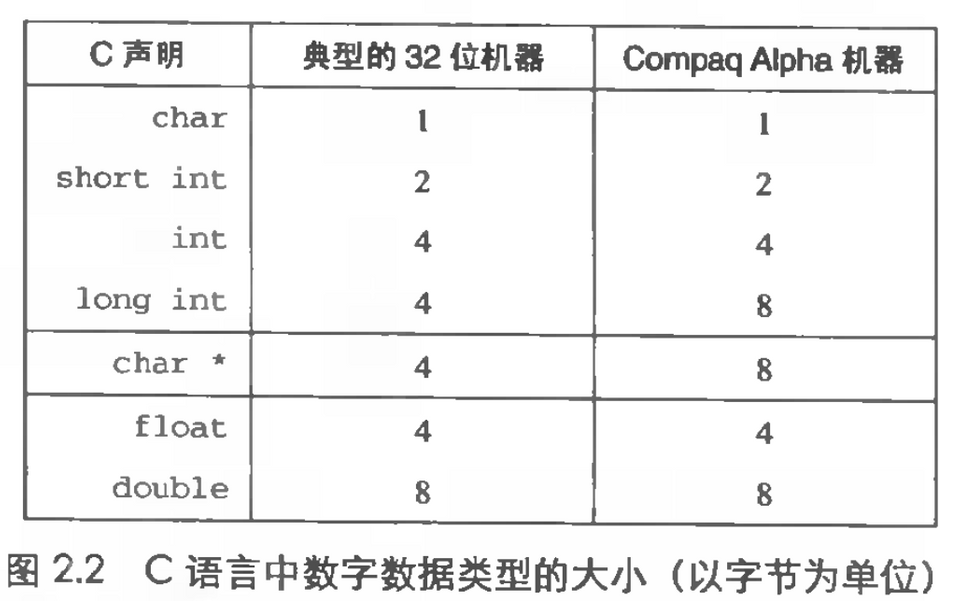### 寻址和字节顺序

int 类型地址在 0x100，存 0x01234567：

1大端法: 0x100 0x101 0x102 0x1032        01    23    45    6734小端法: 0x100 0x101 0x102 0x1035(Intel) 67    45    23    01
byte_pointer.c
1#include <stdio.h>2#include <stdlib.h>34typedef unsigned char *byte_pointer; // 定义类型56void show_bytes(byte_pointer start, int len) {7 int i;8 for (i = 0; i < len; i++)9   printf(" %.2x", start[i]);10  printf("\n");11}1213void show_int(int x) {14  show_bytes((byte_pointer) &x, sizeof(int));15}1617void show_float(float x) {18  show_bytes((byte_pointer) &x, sizeof(float));19}2021void show_pointer(void *x) {22  show_bytes((byte_pointer) &x, sizeof(void *));23}2425int main(int argc, char *argv[]) { // argc 命令行参数个数，argv 命令行参数26  printf(" %s\n", argv);2728  int int_value = atoi(argv); // char * 转 int29  float float_value = (float) int_value; // 强制类型转换30  int *pointer_value = &int_value;3132  show_int(int_value);33  show_float(float_value);34  show_pointer(pointer_value);3536  return 0;37}
1$gcc byte_pointer.c -o byte_pointer2$ byte_pointer 123453 123454 39 30 00 005 00 e4 40 466 7c 32 6d e2 fe 7f 00 00

int 12345 十六进制是 0x00003039，float 12345 表示为 0x4640E400，其中有 13 位相匹配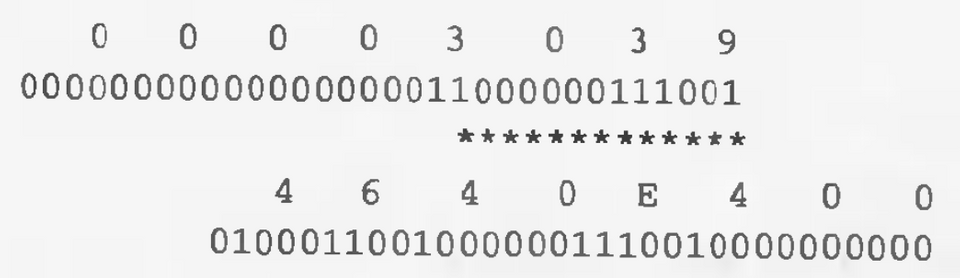### 表示字符串

C 中字符串以 null（\0）结尾，ASCII 码中 0x00 表示 \0，十进制数字 n 由 0x3n 表示，A～Z 由 0x41～0x5A 表示，man ascii 可以得到 ASCII 表

1char *s = "ABCDEF";2show_bytes((byte_pointer) s, strlen(s));3// 41 42 43 44 45 46

ASCII 只适用于编码英语，编码其他特殊字符和语言需要 Unicode

### 布尔代数和环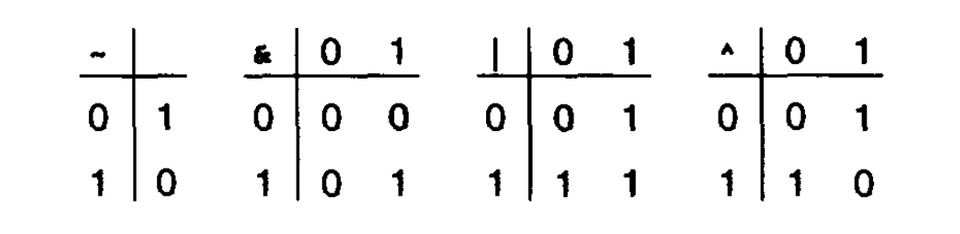a01101001
b01010101
~a10010110
~b10101010
a&b01000001
a|b01111101
a^b00111100

### C 中的位级运算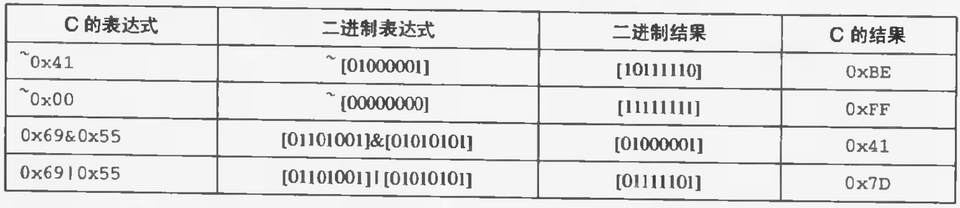1void inplace_swap(int *x, int *y) {2  *x = *x ^ *y;3  *y = *x ^ *y; // *x ^ *y ^ *y == *x4  *x = *x ^ *y; // *x ^ *y ^ *x == *y5}

### C 中的移位运算

xx << 3x >> 2（逻辑）x >> 2（算数）
0xF0 - 111100000x80 - 100000000x3C - 001111000xFC - 11111100

## 整数表示

### 整型数据类型

char: -128 ~ 127 unsigned char: 0 ~ 255 ($2^8$, 1 bytes) short: -32768 ~ 32767 unsigned short: 0 ~ 65535 ($2^{16}$, 2 bytes) int: -2147483648 ~ 2147483647 unsigned int: 0 ~ 4294967295 ($2^{32}$, 4 bytes) long: (4 bytes) long long: (8 bytes)

### 无符号与补码

x无符号补码
0xA - 1010$2^3+2^1=10$$-2^3+2^1=6$
0x8 - 1000$2^3=8$$-2^3=-8$
0xF - 1111$2^3+2^2+2^1+2^0=15$$-2^3+2^2+2^1+2^0=-1$

### 截断数字

1int x = 53191;2short y = (short) x; // -123453int z = y; // -12345

x 对应 0000 0000 0000 0000 1100 1111 1100 0111 截断成 1100 1111 1100 0111，符号位是 1 所以是负的，100 1111 1100 0111 是 12345 的补码（减一取反的原码）

## 整数运算

### 补码的非

5 = 0101 取反 1010 = ($-2^3+1*2^1$) = -6 加一 1011 = -5

### 补码的乘法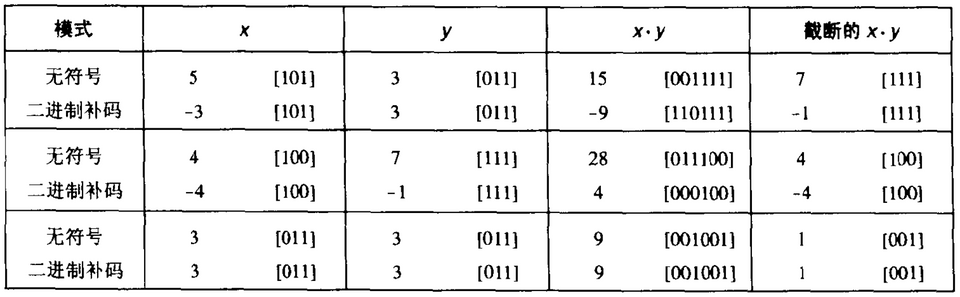## 浮点

### 二进制小数

$12.34_{10}=1*10^1+2+10^0+3*10^{-1}+4*10^{-2}=12\frac{34}{100}$

$101.11_2=1*2^2+0*2^1+1*2^0+1*2^{-1}+1*2^{-2}=4+0+1+\frac{1}{2}+\frac{1}{4}=5\frac{3}{4}$

### IEEE 浮点表示

$value=(-1)^s*M*2^E$

32 位 float：s 占 1 位，exp 占 k=8 位，frac 占 23 位

Bias = $2^{k-1}-1$（32 位是 127，64 位是 1023）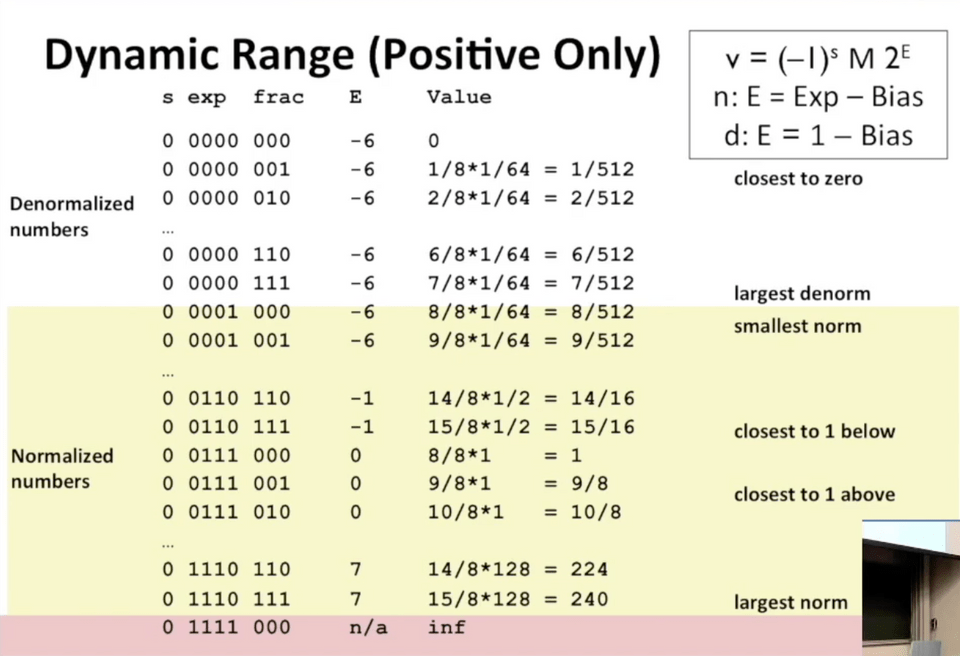### 舍入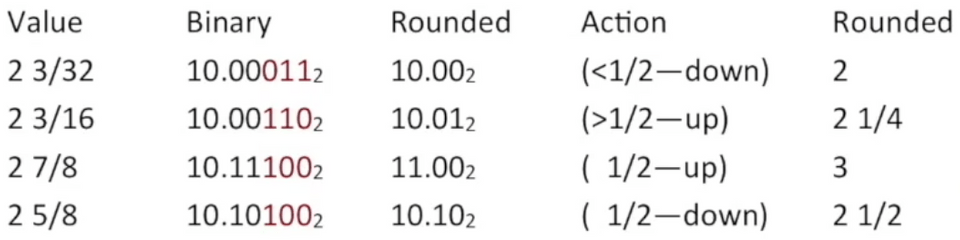## data lab

// TODO: data lab